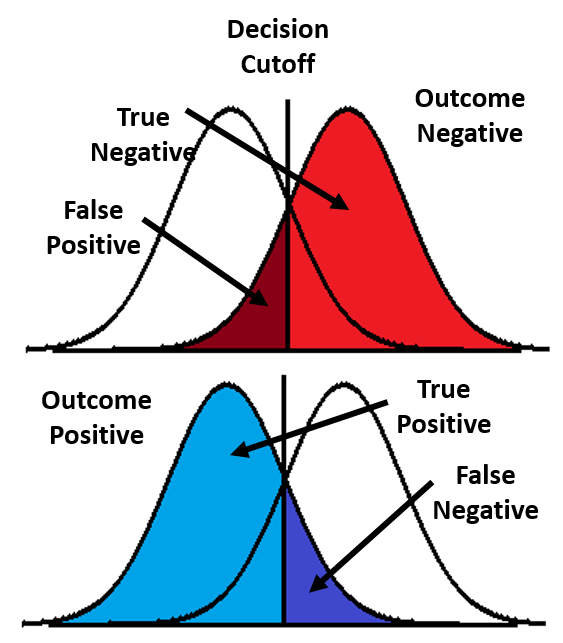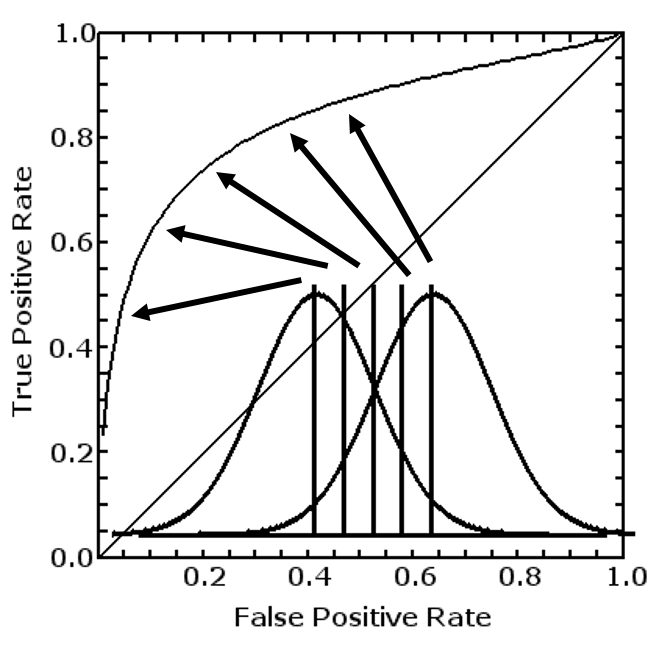Content DisclaimerCopyright @2020.All Rights Reserved.
StatsToDo : Receiver Operator Characteristics (ROCs) Explained
 Introduction Unpaired ROC Paired ROC References StatsToDo offers a suite of programs and explanations related to the statistics predictive tests. As all of them contains a great deal of details, many aspects discussed elsewhere will not be repeated here. This page discusses only Receiver Operator Characteristics (ROC), and supports the program in the Receiver Operator Characteristics (ROC) Program Page . Users are referred to the Prediction Statistics Explanation Page and the Meta-analysis for Predictions Explanation Page for other aspects of prediction, and the Sample Size for Receiver Operator Characteristics (ROC) Explained and Tables Page for sample size required in using the ROC. Historical Aspects : During the Second World War, the British invented the RADAR which reflects a radio wave against an object, and so were able to detect the German bombers coming over the channel. The early versions of RADAR was imprecise, and the signal contains much electronic noise and difficult to interpret. If the alarm was raised with a weak signal, there was a risk of false signal, wasting much fuel and energy in response. If the alarm was not raised until a clear signal is obtained, the risk was that the fighters were scrambled too late and more bombs would be dropped on the cities. In order to analyse the signal and make decisions on how to respond, the scientist developed a statistical method of handling the signals, and named this the RADAR Receiver Operator Characteristics. The method became widely used in signal analysis in many aspects of science and industry, and adopted in the analysis of tests and diagnosis in Medicine, under the term Receiver Operator Characteristics (ROC), with the RADAR part removed.Theoretical Considerations : A scalar, preferably normally distributed measurement is used as a Test to predict a binary Outcome. We will use the use of maternal height (Test) to predict the need for Caesarean Section to deliver the baby (Outcome). The relationship between Test and Outcome is best demonstrated as the diagram to the right. In a sample that was delivered without Caesarean Section (Outcome Negative O-, red), the normal distribution curve defines the height we will use to make a binary decision whether it is Test Positive or Negative. Values of Test less than the decision line (shorter women, maroon) represents the number of False Positives (FP) Values of Test greater than the decision line (taller women, red), represents the True Negatives (TN) True Negative Rate TNR = TN / (TN+FP), and False Positive Rate FPR = FP / (TN+FP) = 1-TNR In a sample that was delivered by Caesarean Section (Outcome Positive O+, blue), the normal distribution curve defines the height we will use to make a binary decision whether it is Test Positive or Negative. Value of Test less than the decision line (shorter women, blue) represents the number of True Positives (TP) Value of Test greater than the decision line (taller women, navy), represents the false negatives (FN) True Posive Rate TPR = TP / (TP+FN), and False Negative Rate FNR = FN / (TP+FN) = 1-TPRIf the value used to make the decision changes, in our example, if the maternal height we used to predict changes, then TP,FP,FN, and TN also changed, and the TPR and FPR changed accordingly. The changing relationship between FPR and TPR forms a curve, as shown in the diagram to the left. This curve is called the Receiver Operator Characteristics Curve (ROC Curve), and the area under this curve is called the Area Under the ROC usually represented by the abbreciation θ In a perfect test, where the values of the test from Outcome Positives do not overlap those from Outcome Negatives, TPR will increase from 0 to 1 while FPR remains at 0. TPR will then remain at 1 while FPR increases from 0 to 1. In other words, the ROC curve hugs the left and top border, and the area under it θ=1 In a completely useless test, the values of measurement from Outcome Positives and Outcome Negatives overlap completely, so that the TPR and FPR increase together. The ROC curve is therefore the diagonal, and the Area under it θ=0.5 In most cases, there is partial overlap of values, and the ROC curve looks pretty much like that in the diagram above and to the left, with a value between 0.5 and 1 The null value for θ is therefore 0.5, a useful test has a 95% confidence interval of θ that is greater than 0.5. Where θ<0.5, the test predicts the opposite of the intended outcome.